# T-Test of Two Mutually Exclusive Proportions

Where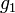$g_1$ and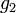$g_2$ are the two proportions: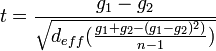$t=\frac{g_1-g_2}{\sqrt{d_{eff}(\frac{g_1+g_2 - (g_1-g_2)^2)}{n - 1})}}$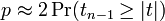$p \approx 2\Pr(t_{n-1} \ge |t|)$,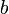$b$ is 1 if Bessel's correction for Means is selected in Statistical Assumptions and 0 otherwise,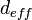$d_{eff}$ is Extra deff, and$n$ is the unweighted sample size.